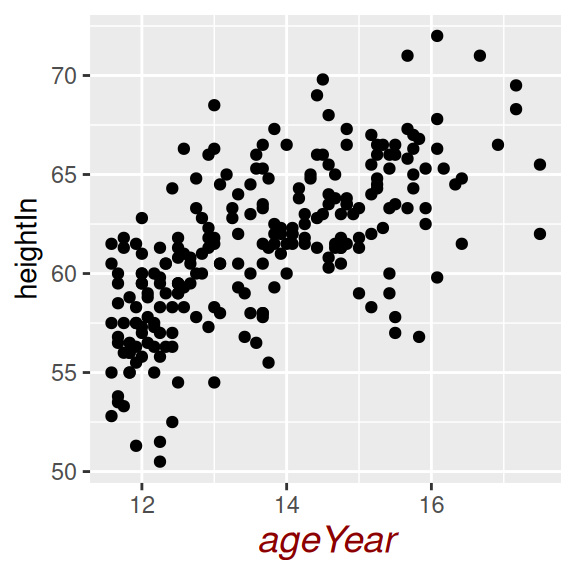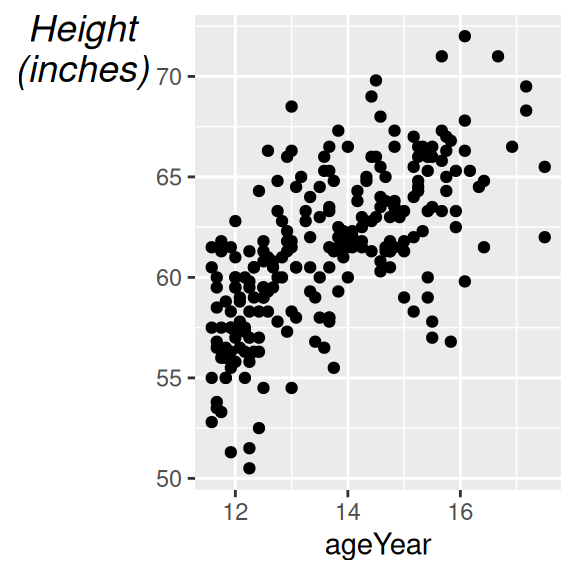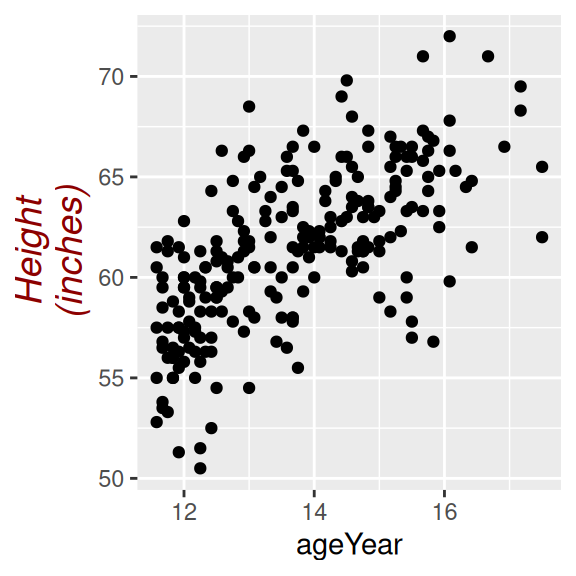## 8.12 Changing the Appearance of Axis Labels

### 8.12.1 Problem

You want to change the appearance of axis labels.

### 8.12.2 Solution

To change the appearance of the x-axis label (Figure 8.22), use `axis.title.x`:

``````library(gcookbook)  # Load gcookbook for the heightweight data set

hw_plot <- ggplot(heightweight, aes(x = ageYear, y = heightIn)) +
geom_point()

hw_plot +
theme(axis.title.x = element_text(face = "italic", colour = "darkred", size = 14))``````Figure 8.22: X-axis label with customized appearance

### 8.12.3 Discussion

For the y-axis label, it might also be useful to display the text unrotated, as shown in Figure 8.23 (left). The `\n` in the label represents a newline character:

``````hw_plot +
ylab("Height\n(inches)") +
theme(axis.title.y = element_text(angle = 0, face = "italic", size = 14))``````

When you call `element_text()`, the default `angle` is 0, so if you set `axis.title.y` but don’t specify the `angle`, it will show in this orientation, with the top of the text pointing up. If you change any other properties of `axis.title.y` and want it to be displayed in its usual orientation, rotated 90 degrees, you must manually specify the `angle` (Figure 8.23, right):

``````hw_plot +
ylab("Height\n(inches)") +
theme(axis.title.y = element_text(
angle = 90,
face = "italic",
colour = "darkred",
size = 14)
)``````Figure 8.23: Y-axis label with angle = 0 (left); With angle = 90 (right)